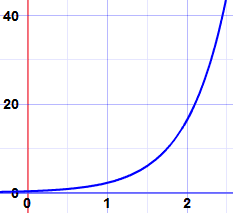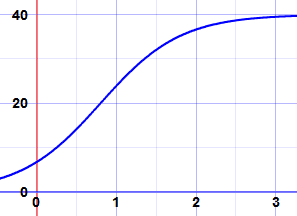# 分离变量法## 什么时候可以用？## 方法

• 一、把所有 y 项（包括 dy）移到方程的一边，把所有 x 项（包括 dx）移到另一边。
• 二、把一边对 y 积分，另一边对 x 积分。不要忘了 "+ C" （积分常数）。
• 三、简化

### 例子：解（k 是常数）

dy dx = ky

 每边乘以 dx： dy = ky dx 每边除以 y： dy y = k dx

 积分符号放在前面： ∫ dy y = ∫k dx 求左边的积分： ln(y) + C = ∫k dx 求右边的积分： ln(y) + C = kx + D

C 是积分常数。用 D 来代表另一个（不同的）积分常数。

 合并两个常数为一个(a=D−C): ln(y) = kx + a e(ln(y)) = y，所以我们取每边的幂： y = ekx + a ekx + a = ekx ea，所以这是： y = ekx ea ea 是个常数，我们用 c 来代替它 y = cekx

y = cekx### 例子：兔子！

• 在任何时间 t 时兔子的数量 N
• 增长率 r
• 数量变化率 dN dt

dN dt = rN

• 是 N， 不是 y
• 是 t， 不是 x
• 是 r， 不是 k

N = cert## 更多例子

### 例子：解

 dy = 1 dx y

 每边乘以 dx： dy = (1/y) dx 每边乘以 y： y dy = dx

 放积分符号在前面： ∫y dy = ∫dx 每边求积分： (y2)/2 = x + C

 每边乘以 2： y2 = 2(x + C) 每边取平方根： y = ±√(2(x + C))

y = ±√(2(x + C))

### 例子：解

 dy = 2xy dx 1 + x2

 1 dy  = 2x dx y 1 + x2

 ∫ 1 dy  = ∫ 2x dx y 1 + x2

u = 1 + x2，所以 du = 2x dx
 ∫ 1 dy  = ∫ 1 du y u

C = ln(k)   ln(y) = ln(u) + ln(k)

y = k(1 + x2)### 例子：又是兔子！

 dN = rN dt

 dN = rN(1-N/k) dt

 1 dN = r dt N(1−N/k)

 ∫ 1 dN  = ∫r dt N(1−N/k)

 1 N(1−N/k)

 k N(k−N)

（去看看 部分分式）：

 N+k−N N(k−N)

 N + k−N N(k−N) N(k−N)

 1 + 1 k−N N

 ∫ 1 dN + ∫ 1 dN  = ∫r dt k−N N

（为什么变成 ln(k−N）？因为我们是对 N 求积分。）

 取所有项的负值： ln(k−N) − ln(N) = −rt − C 合并 ln()： ln((k−N)/N) = −rt − C 取每边的幂： (k−N)/N = e−rt−C 分离 e 的幂： (k−N)/N = e−rt e−C e−C 是常数，我们可以用 A 来代替它： (k−N)/N = Ae−rt

 分离分数项： (k/N)−1 = Ae−rt 每边加 1： k/N = 1 + Ae−rt 除以 k： 1/N = (1 + Ae−rt)/k 每边取倒数： N = k/(1 + Ae−rt)

 N = k 1 + Ae−rt

 举个例，这是 40 的图： 1 + 5e−2t